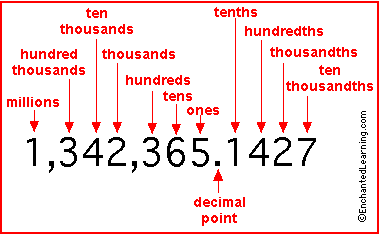# What Is 10 To The Power Of Minus 3 =?

For each smaller power of 10, you put the digit one decimal place to the right.
102 = 100.
101 = 10.
100 = 1.
10-1 = .1
10-2 = .01
10-3 = .001thanked the writer.